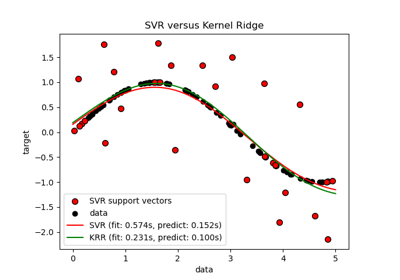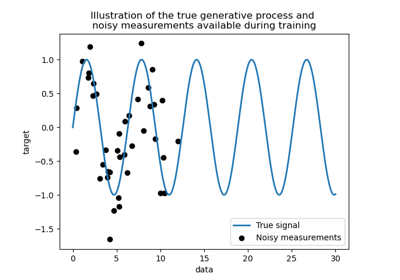# sklearn.kernel_ridge.KernelRidge¶

class sklearn.kernel_ridge.KernelRidge(alpha=1, kernel='linear', gamma=None, degree=3, coef0=1, kernel_params=None)[source]

Kernel ridge regression.

Kernel ridge regression (KRR) combines ridge regression (linear least squares with l2-norm regularization) with the kernel trick. It thus learns a linear function in the space induced by the respective kernel and the data. For non-linear kernels, this corresponds to a non-linear function in the original space.

The form of the model learned by KRR is identical to support vector regression (SVR). However, different loss functions are used: KRR uses squared error loss while support vector regression uses epsilon-insensitive loss, both combined with l2 regularization. In contrast to SVR, fitting a KRR model can be done in closed-form and is typically faster for medium-sized datasets. On the other hand, the learned model is non-sparse and thus slower than SVR, which learns a sparse model for epsilon > 0, at prediction-time.

This estimator has built-in support for multi-variate regression (i.e., when y is a 2d-array of shape [n_samples, n_targets]).

Read more in the User Guide.

Parameters
alpha{float, array-like}, shape = [n_targets]

Small positive values of alpha improve the conditioning of the problem and reduce the variance of the estimates. Alpha corresponds to (2*C)^-1 in other linear models such as LogisticRegression or LinearSVC. If an array is passed, penalties are assumed to be specific to the targets. Hence they must correspond in number.

kernelstring or callable, default=”linear”

Kernel mapping used internally. A callable should accept two arguments and the keyword arguments passed to this object as kernel_params, and should return a floating point number. Set to “precomputed” in order to pass a precomputed kernel matrix to the estimator methods instead of samples.

gammafloat, default=None

Gamma parameter for the RBF, laplacian, polynomial, exponential chi2 and sigmoid kernels. Interpretation of the default value is left to the kernel; see the documentation for sklearn.metrics.pairwise. Ignored by other kernels.

degreefloat, default=3

Degree of the polynomial kernel. Ignored by other kernels.

coef0float, default=1

Zero coefficient for polynomial and sigmoid kernels. Ignored by other kernels.

kernel_paramsmapping of string to any, optional

Additional parameters (keyword arguments) for kernel function passed as callable object.

Attributes
dual_coef_array, shape = [n_samples] or [n_samples, n_targets]

Representation of weight vector(s) in kernel space

X_fit_{array-like, sparse matrix} of shape (n_samples, n_features)

Training data, which is also required for prediction. If kernel == “precomputed” this is instead the precomputed training matrix, shape = [n_samples, n_samples].

sklearn.linear_model.Ridge

Linear ridge regression.

sklearn.svm.SVR

Support Vector Regression implemented using libsvm.

References

• Kevin P. Murphy “Machine Learning: A Probabilistic Perspective”, The MIT Press chapter 14.4.3, pp. 492-493

Examples

>>> from sklearn.kernel_ridge import KernelRidge
>>> import numpy as np
>>> n_samples, n_features = 10, 5
>>> rng = np.random.RandomState(0)
>>> y = rng.randn(n_samples)
>>> X = rng.randn(n_samples, n_features)
>>> clf = KernelRidge(alpha=1.0)
>>> clf.fit(X, y)
KernelRidge(alpha=1.0)


Methods

 fit(self, X[, y, sample_weight]) Fit Kernel Ridge regression model get_params(self[, deep]) Get parameters for this estimator. predict(self, X) Predict using the kernel ridge model score(self, X, y[, sample_weight]) Return the coefficient of determination R^2 of the prediction. set_params(self, \*\*params) Set the parameters of this estimator.
__init__(self, alpha=1, kernel='linear', gamma=None, degree=3, coef0=1, kernel_params=None)[source]

Initialize self. See help(type(self)) for accurate signature.

fit(self, X, y=None, sample_weight=None)[source]

Fit Kernel Ridge regression model

Parameters
X{array-like, sparse matrix} of shape (n_samples, n_features)

Training data. If kernel == “precomputed” this is instead a precomputed kernel matrix, shape = [n_samples, n_samples].

yarray-like of shape (n_samples,) or (n_samples, n_targets)

Target values

sample_weightfloat or array-like of shape [n_samples]

Individual weights for each sample, ignored if None is passed.

Returns
selfreturns an instance of self.
get_params(self, deep=True)[source]

Get parameters for this estimator.

Parameters
deepbool, default=True

If True, will return the parameters for this estimator and contained subobjects that are estimators.

Returns
paramsmapping of string to any

Parameter names mapped to their values.

predict(self, X)[source]

Predict using the kernel ridge model

Parameters
X{array-like, sparse matrix} of shape (n_samples, n_features)

Samples. If kernel == “precomputed” this is instead a precomputed kernel matrix, shape = [n_samples, n_samples_fitted], where n_samples_fitted is the number of samples used in the fitting for this estimator.

Returns
Cndarray of shape (n_samples,) or (n_samples, n_targets)

Returns predicted values.

score(self, X, y, sample_weight=None)[source]

Return the coefficient of determination R^2 of the prediction.

The coefficient R^2 is defined as (1 - u/v), where u is the residual sum of squares ((y_true - y_pred) ** 2).sum() and v is the total sum of squares ((y_true - y_true.mean()) ** 2).sum(). The best possible score is 1.0 and it can be negative (because the model can be arbitrarily worse). A constant model that always predicts the expected value of y, disregarding the input features, would get a R^2 score of 0.0.

Parameters
Xarray-like of shape (n_samples, n_features)

Test samples. For some estimators this may be a precomputed kernel matrix or a list of generic objects instead, shape = (n_samples, n_samples_fitted), where n_samples_fitted is the number of samples used in the fitting for the estimator.

yarray-like of shape (n_samples,) or (n_samples, n_outputs)

True values for X.

sample_weightarray-like of shape (n_samples,), default=None

Sample weights.

Returns
scorefloat

R^2 of self.predict(X) wrt. y.

Notes

The R2 score used when calling score on a regressor will use multioutput='uniform_average' from version 0.23 to keep consistent with r2_score. This will influence the score method of all the multioutput regressors (except for MultiOutputRegressor). To specify the default value manually and avoid the warning, please either call r2_score directly or make a custom scorer with make_scorer (the built-in scorer 'r2' uses multioutput='uniform_average').

set_params(self, **params)[source]

Set the parameters of this estimator.

The method works on simple estimators as well as on nested objects (such as pipelines). The latter have parameters of the form <component>__<parameter> so that it’s possible to update each component of a nested object.

Parameters
**paramsdict

Estimator parameters.

Returns
selfobject

Estimator instance.

## Examples using sklearn.kernel_ridge.KernelRidge¶